# Topological Sorting

Topological sorting for Directed Acyclic Graph (DAG) is a linear ordering of vertices such that for every directed edge u-v, vertex u comes before v in the ordering.

Note: Topological Sorting for a graph is not possible if the graph is not a DAG.

Example:

Input: Graph :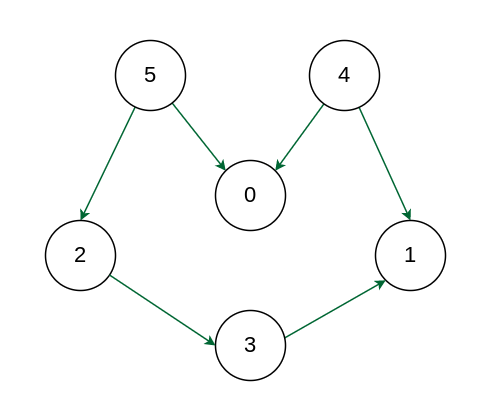Example

Output: 5 4 2 3 1 0
Explanation: The first vertex in topological sorting is always a vertex with an in-degree of 0 (a vertex with no incoming edges).  A topological sorting of the following graph is “5 4 2 3 1 0”. There can be more than one topological sorting for a graph. Another topological sorting of the following graph is “4 5 2 3 1 0”.

Recommended Practice

## Topological Sortingvs Depth First Traversal (DFS):

In DFS, we print a vertex and then recursively call DFS for its adjacent vertices. In topological sorting, we need to print a vertex before its adjacent vertices.

For example, In the above given graph, the vertex ‘5’ should be printed before vertex ‘0’, but unlike DFS, the vertex ‘4’ should also be printed before vertex ‘0’. So Topological sorting is different from DFS. For example, a DFS of the shown graph is “5 2 3 1 0 4”, but it is not a topological sorting.

## Algorithm for Topological Sorting:

Prerequisite: DFS

We can modify DFS to find the Topological Sorting of a graph. In DFS

• We start from a vertex, we first print it, and then
• Recursively call DFS for its adjacent vertices.

In topological sorting:

• We use a temporary stack.
• We don’t print the vertex immediately,
• We first recursively call topological sorting for all its adjacent vertices, then push it to a stack.
• Finally, print the contents of the stack.

Note: A vertex is pushed to stack only when all of its adjacent vertices (and their adjacent vertices and so on) are already in the stack

Approach:

• Create a stack to store the nodes.
• Initialize visited array of size N to keep the record of visited nodes.
• Run a loop from 0 till N :
• if the node is not marked True in visited array then call the recursive function for topological sort and perform the following steps:
• Mark the current node as True in the visited array.
• Run a loop on all the nodes which has a directed edge to the current node
• if the node is not marked True in the visited array:
• Recursively call the topological sort function on the node
• Push the current node in the stack.
• Print all the elements in the stack.

### Illustration Topological Sorting Algorithm:

Below image is an illustration of the above approach: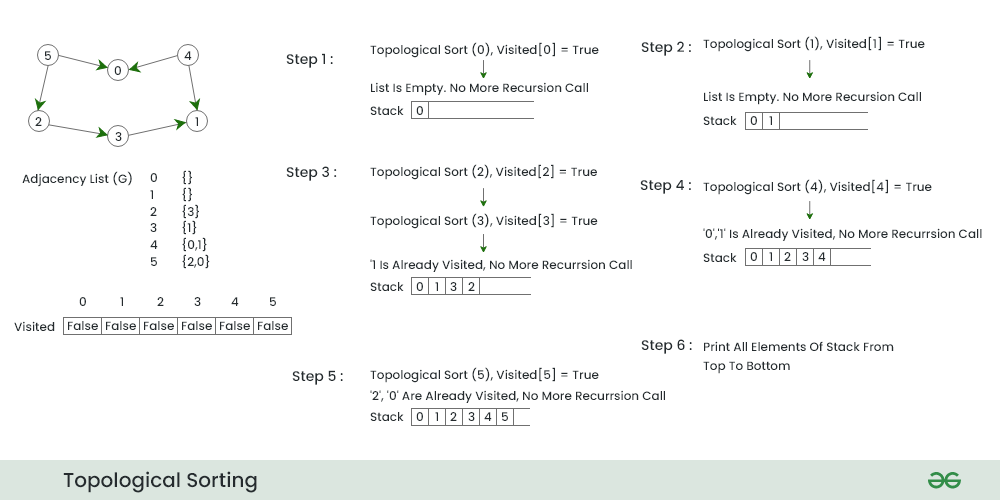Overall workflow of topological sorting

Step1:

• We start DFS from node 0 because it has zero incoming Nodes
• We push node 0 in the stack and move to next node having minimum number of adjacent nodes i.e. node 1.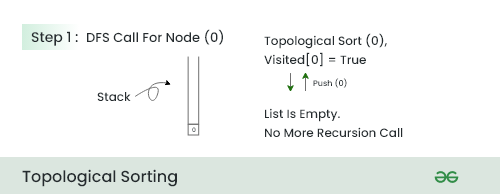Step 2:

• In this step , because there is no adjacent of this node so push the node 1 in the stack and move to next node.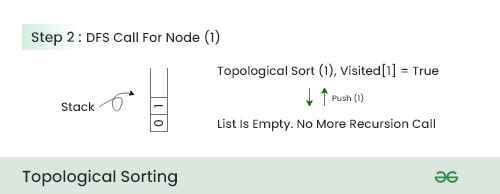Step 3:

• In this step , We choose node 2 because it has minimum number of adjacent nodes after 0 and 1 .
• We call DFS for node 2 and push all the nodes which comes in traversal from node 2 in reverse order.
• So push 3 then push 2 .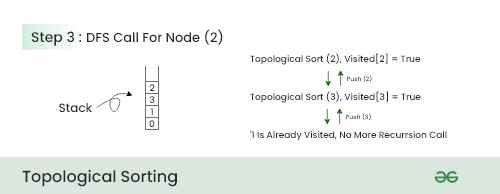Step 4:

• We now call DFS for node 4
• Because 0 and 1 already present in the stack so we just push node 4 in the stack and return.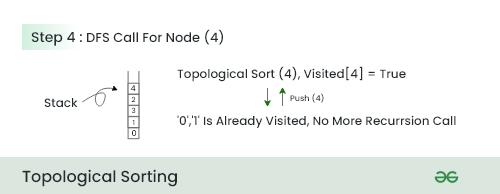Step 5:

• In this step because all the adjacent nodes of 5 is already in the stack we push node 5 in the stack and return.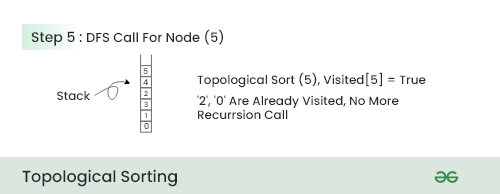Step 6: This is the final step of the Topological sorting in which we pop all the element from the stack and print it in that order .

Below is the implementation of the above approach:

## C++

 `// A C++ program to print topological` `// sorting of a DAG` `#include ` `using` `namespace` `std;`   `// Class to represent a graph` `class` `Graph {` `    ``// No. of vertices'` `    ``int` `V;`   `    ``// Pointer to an array containing adjacency listsList` `    ``list<``int``>* adj;`   `    ``// A function used by topologicalSort` `    ``void` `topologicalSortUtil(``int` `v, ``bool` `visited[],` `                             ``stack<``int``>& Stack);`   `public``:` `    ``// Constructor` `    ``Graph(``int` `V);`   `    ``// function to add an edge to graph` `    ``void` `addEdge(``int` `v, ``int` `w);`   `    ``// prints a Topological Sort of` `    ``// the complete graph` `    ``void` `topologicalSort();` `};`   `Graph::Graph(``int` `V)` `{` `    ``this``->V = V;` `    ``adj = ``new` `list<``int``>[V];` `}`   `void` `Graph::addEdge(``int` `v, ``int` `w)` `{` `    ``// Add w to v’s list.` `    ``adj[v].push_back(w);` `}`   `// A recursive function used by topologicalSort` `void` `Graph::topologicalSortUtil(``int` `v, ``bool` `visited[],` `                                ``stack<``int``>& Stack)` `{` `    ``// Mark the current node as visited.` `    ``visited[v] = ``true``;`   `    ``// Recur for all the vertices` `    ``// adjacent to this vertex` `    ``list<``int``>::iterator i;` `    ``for` `(i = adj[v].begin(); i != adj[v].end(); ++i)` `        ``if` `(!visited[*i])` `            ``topologicalSortUtil(*i, visited, Stack);`   `    ``// Push current vertex to stack` `    ``// which stores result` `    ``Stack.push(v);` `}`   `// The function to do Topological Sort.` `// It uses recursive topologicalSortUtil()` `void` `Graph::topologicalSort()` `{` `    ``stack<``int``> Stack;`   `    ``// Mark all the vertices as not visited` `    ``bool``* visited = ``new` `bool``[V];` `    ``for` `(``int` `i = 0; i < V; i++)` `        ``visited[i] = ``false``;`   `    ``// Call the recursive helper function` `    ``// to store Topological` `    ``// Sort starting from all` `    ``// vertices one by one` `    ``for` `(``int` `i = 0; i < V; i++)` `        ``if` `(visited[i] == ``false``)` `            ``topologicalSortUtil(i, visited, Stack);`   `    ``// Print contents of stack` `    ``while` `(Stack.empty() == ``false``) {` `        ``cout << Stack.top() << ``" "``;` `        ``Stack.pop();` `    ``}`   `    ``delete``[] visited;` `}`   `// Driver Code` `int` `main()` `{` `    ``// Create a graph given in the above diagram` `    ``Graph g(6);` `    ``g.addEdge(5, 2);` `    ``g.addEdge(5, 0);` `    ``g.addEdge(4, 0);` `    ``g.addEdge(4, 1);` `    ``g.addEdge(2, 3);` `    ``g.addEdge(3, 1);`   `    ``cout << ``"Following is a Topological Sort of the given "` `            ``"graph \n"``;`   `    ``// Function Call` `    ``g.topologicalSort();`   `    ``return` `0;` `}`

## Java

 `// A Java program to print topological` `// sorting of a DAG` `import` `java.io.*;` `import` `java.util.*;`   `// This class represents a directed graph` `// using adjacency list representation` `class` `Graph {` `    ``// No. of vertices` `    ``private` `int` `V;`   `    ``// Adjacency List as ArrayList of ArrayList's` `    ``private` `ArrayList > adj;`   `    ``// Constructor` `    ``Graph(``int` `v)` `    ``{` `        ``V = v;` `        ``adj = ``new` `ArrayList >(v);` `        ``for` `(``int` `i = ``0``; i < v; ++i)` `            ``adj.add(``new` `ArrayList());` `    ``}`   `    ``// Function to add an edge into the graph` `    ``void` `addEdge(``int` `v, ``int` `w) { adj.get(v).add(w); }`   `    ``// A recursive function used by topologicalSort` `    ``void` `topologicalSortUtil(``int` `v, ``boolean` `visited[],` `                             ``Stack stack)` `    ``{` `        ``// Mark the current node as visited.` `        ``visited[v] = ``true``;` `        ``Integer i;`   `        ``// Recur for all the vertices adjacent` `        ``// to thisvertex` `        ``Iterator it = adj.get(v).iterator();` `        ``while` `(it.hasNext()) {` `            ``i = it.next();` `            ``if` `(!visited[i])` `                ``topologicalSortUtil(i, visited, stack);` `        ``}`   `        ``// Push current vertex to stack` `        ``// which stores result` `        ``stack.push(``new` `Integer(v));` `    ``}`   `    ``// The function to do Topological Sort.` `    ``// It uses recursive topologicalSortUtil()` `    ``void` `topologicalSort()` `    ``{` `        ``Stack stack = ``new` `Stack();`   `        ``// Mark all the vertices as not visited` `        ``boolean` `visited[] = ``new` `boolean``[V];` `        ``for` `(``int` `i = ``0``; i < V; i++)` `            ``visited[i] = ``false``;`   `        ``// Call the recursive helper` `        ``// function to store` `        ``// Topological Sort starting` `        ``// from all vertices one by one` `        ``for` `(``int` `i = ``0``; i < V; i++)` `            ``if` `(visited[i] == ``false``)` `                ``topologicalSortUtil(i, visited, stack);`   `        ``// Print contents of stack` `        ``while` `(stack.empty() == ``false``)` `            ``System.out.print(stack.pop() + ``" "``);` `    ``}`   `    ``// Driver code` `    ``public` `static` `void` `main(String args[])` `    ``{` `        ``// Create a graph given in the above diagram` `        ``Graph g = ``new` `Graph(``6``);` `        ``g.addEdge(``5``, ``2``);` `        ``g.addEdge(``5``, ``0``);` `        ``g.addEdge(``4``, ``0``);` `        ``g.addEdge(``4``, ``1``);` `        ``g.addEdge(``2``, ``3``);` `        ``g.addEdge(``3``, ``1``);`   `        ``System.out.println(``"Following is a Topological "` `                           ``+ ``"sort of the given graph"``);` `        ``// Function Call` `        ``g.topologicalSort();` `    ``}` `}` `// This code is contributed by Aakash Hasija`

## Python3

 `# Python program to print topological sorting of a DAG` `from` `collections ``import` `defaultdict`   `# Class to represent a graph`     `class` `Graph:` `    ``def` `__init__(``self``, vertices):` `        ``self``.graph ``=` `defaultdict(``list``)  ``# dictionary containing adjacency List` `        ``self``.V ``=` `vertices  ``# No. of vertices`   `    ``# function to add an edge to graph` `    ``def` `addEdge(``self``, u, v):` `        ``self``.graph[u].append(v)`   `    ``# A recursive function used by topologicalSort` `    ``def` `topologicalSortUtil(``self``, v, visited, stack):`   `        ``# Mark the current node as visited.` `        ``visited[v] ``=` `True`   `        ``# Recur for all the vertices adjacent to this vertex` `        ``for` `i ``in` `self``.graph[v]:` `            ``if` `visited[i] ``=``=` `False``:` `                ``self``.topologicalSortUtil(i, visited, stack)`   `        ``# Push current vertex to stack which stores result` `        ``stack.append(v)`   `    ``# The function to do Topological Sort. It uses recursive` `    ``# topologicalSortUtil()` `    ``def` `topologicalSort(``self``):` `        ``# Mark all the vertices as not visited` `        ``visited ``=` `[``False``]``*``self``.V` `        ``stack ``=` `[]`   `        ``# Call the recursive helper function to store Topological` `        ``# Sort starting from all vertices one by one` `        ``for` `i ``in` `range``(``self``.V):` `            ``if` `visited[i] ``=``=` `False``:` `                ``self``.topologicalSortUtil(i, visited, stack)`   `        ``# Print contents of the stack` `        ``print``(stack[::``-``1``])  ``# return list in reverse order`     `# Driver Code` `if` `__name__ ``=``=` `'__main__'``:` `    ``g ``=` `Graph(``6``)` `    ``g.addEdge(``5``, ``2``)` `    ``g.addEdge(``5``, ``0``)` `    ``g.addEdge(``4``, ``0``)` `    ``g.addEdge(``4``, ``1``)` `    ``g.addEdge(``2``, ``3``)` `    ``g.addEdge(``3``, ``1``)`   `    ``print``(``"Following is a Topological Sort of the given graph"``)`   `    ``# Function Call` `    ``g.topologicalSort()`   `# This code is contributed by Neelam Yadav`

## C#

 `using` `System;` `using` `System.Collections.Generic;`   `// Class to represent a graph` `class` `Graph` `{` `    ``// No. of vertices` `    ``private` `int` `V;`   `    ``// Array containing adjacency lists` `    ``private` `List<``int``>[] adj;`   `    ``// Constructor` `    ``public` `Graph(``int` `V)` `    ``{` `        ``this``.V = V;` `        ``adj = ``new` `List<``int``>[V];` `        ``for` `(``int` `i = 0; i < V; i++)` `        ``{` `            ``adj[i] = ``new` `List<``int``>();` `        ``}` `    ``}`   `    ``// Function to add an edge to graph` `    ``public` `void` `AddEdge(``int` `v, ``int` `w)` `    ``{` `        ``// Add w to v's list` `        ``adj[v].Add(w);` `    ``}`   `    ``// A recursive function used by TopologicalSort` `    ``private` `void` `TopologicalSortUtil(``int` `v, ``bool``[] visited, Stack<``int``> stack)` `    ``{` `        ``// Mark the current node as visited` `        ``visited[v] = ``true``;`   `        ``// Recur for all the vertices adjacent to this vertex` `        ``foreach` `(``var` `i ``in` `adj[v])` `        ``{` `            ``if` `(!visited[i])` `            ``{` `                ``TopologicalSortUtil(i, visited, stack);` `            ``}` `        ``}`   `        ``// Push current vertex to stack which stores result` `        ``stack.Push(v);` `    ``}`   `    ``// The function to do Topological Sort. It uses recursive TopologicalSortUtil` `    ``public` `void` `TopologicalSort()` `    ``{` `        ``Stack<``int``> stack = ``new` `Stack<``int``>();`   `        ``// Mark all the vertices as not visited` `        ``bool``[] visited = ``new` `bool``[V];` `        ``for` `(``int` `i = 0; i < V; i++)` `        ``{` `            ``visited[i] = ``false``;` `        ``}`   `        ``// Call the recursive helper function to store Topological Sort starting from all vertices one by one` `        ``for` `(``int` `i = 0; i < V; i++)` `        ``{` `            ``if` `(!visited[i])` `            ``{` `                ``TopologicalSortUtil(i, visited, stack);` `            ``}` `        ``}`   `        ``// Print contents of stack` `        ``Console.Write(``"Following is a Topological Sort of the given graph: "``);` `        ``while` `(stack.Count > 0)` `        ``{` `            ``Console.Write(stack.Pop() + ``" "``);` `        ``}` `        ``Console.WriteLine(); ``// Add a new line for better formatting` `    ``}` `}`   `// Driver Code` `class` `Program` `{` `    ``static` `void` `Main()` `    ``{` `        ``// Create a graph given in the above diagram` `        ``Graph g = ``new` `Graph(6);` `        ``g.AddEdge(5, 2);` `        ``g.AddEdge(5, 0);` `        ``g.AddEdge(4, 0);` `        ``g.AddEdge(4, 1);` `        ``g.AddEdge(2, 3);` `        ``g.AddEdge(3, 1);`   `        ``Console.WriteLine(``""``);`   `        ``// Function Call` `        ``g.TopologicalSort();` `    ``}` `}`

## Javascript

 `// Javascript for the above approach`   `    ``// This class represents a directed graph` `    ``// using adjacency list representation` `    ``class Graph{`   `        ``// Constructor` `        ``constructor(v)` `        ``{` `            ``// Number of vertices` `            ``this``.V = v`   `            ``// Adjacency List as ArrayList of ArrayList's` `            ``this``.adj = ``new` `Array(``this``.V)` `            ``for` `(let i = 0 ; i < ``this``.V ; i+=1){` `                ``this``.adj[i] = ``new` `Array()` `            ``}` `        ``}`   `        ``// Function to add an edge into the graph` `        ``addEdge(v, w){` `            ``this``.adj[v].push(w)` `        ``}`   `        ``// A recursive function used by topologicalSort` `        ``topologicalSortUtil(v, visited, stack)` `        ``{` `            ``// Mark the current node as visited.` `            ``visited[v] = ``true``;` `            ``let i = 0;`   `            ``// Recur for all the vertices adjacent` `            ``// to thisvertex` `            ``for``(i = 0 ; i < ``this``.adj[v].length ; i++){` `                ``if``(!visited[``this``.adj[v][i]]){` `                    ``this``.topologicalSortUtil(``this``.adj[v][i], visited, stack)` `                ``}` `            ``}`   `            ``// Push current vertex to stack` `            ``// which stores result` `            ``stack.push(v);` `        ``}`   `        ``// The function to do Topological Sort.` `        ``// It uses recursive topologicalSortUtil()` `        ``topologicalSort()` `        ``{` `            ``let stack = ``new` `Array()`   `            ``// Mark all the vertices as not visited` `            ``let visited = ``new` `Array(``this``.V);` `            ``for` `(let i = 0 ; i < ``this``.V ; i++){` `                ``visited[i] = ``false``;` `            ``}`   `            ``// Call the recursive helper` `            ``// function to store` `            ``// Topological Sort starting` `            ``// from all vertices one by one` `            ``for` `(let i = 0 ; i < ``this``.V ; i++){` `                ``if` `(visited[i] == ``false``){` `                    ``this``.topologicalSortUtil(i, visited, stack);` `                ``}` `            ``}`   `            ``// Print contents of stack` `            ``while` `(stack.length != 0){` `                ``console.log(stack.pop() + ``" "``)` `            ``}` `        ``}` `    ``}`   `    ``// Driver Code` `    ``var` `g = ``new` `Graph(6)` `    ``g.addEdge(5, 2)` `    ``g.addEdge(5, 0)` `    ``g.addEdge(4, 0)` `    ``g.addEdge(4, 1)` `    ``g.addEdge(2, 3)` `    ``g.addEdge(3, 1)` `    `  `    ``console.log(``"Following is a Topological sort of the given graph"``)` `    `  `    ``// Function Call` `    ``g.topologicalSort()` `    `  `    ``// This code is contributed by subhamgoyal2014.`

Output

```Following is a Topological Sort of the given graph
5 4 2 3 1 0 ```

Time Complexity: O(V+E). The above algorithm is simply DFS with an extra stack. So time complexity is the same as DFS
Auxiliary space: O(V). The extra space is needed for the stack

Note: Here, we can also use a vector instead of the stack. If the vector is used then print the elements in reverse order to get the topological sorting.

## Applications of Topological Sorting:

• Topological Sorting is mainly used for scheduling jobs from the given dependencies among jobs.
• In computer science, applications of this type arise in:
• Instruction scheduling
• Ordering of formula cell evaluation when recomputing formula values in spreadsheets
• Logic synthesis
• Determining the order of compilation tasks to perform in make files
• Data serialization
• Resolving symbol dependencies in linkers

Related Articles: